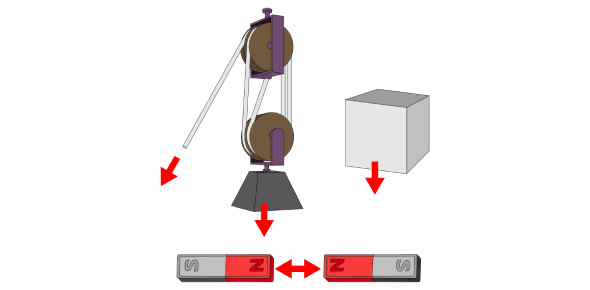# Identifying Forces Quiz

9 Questions | Attempts: 4222
ShareSettingsOnce you have completed this quiz with a score of 90% or greater, get a teacher signature before moving on to the Quiz "Force Calculations"

• 1.
What is a force?
• A.

A push or a pull

• B.

A newton

• C.

The ability to levitate objects like Luke sky-walker

• 2.
Elastic force is force is exerted by a material when it is stretched or compressed
• A.

True

• B.

False

• 3.
Friction acts in a direction __________ to the direction of an object's motion
• A.

Unrelated

• B.

Opposite

• C.

Equal

• D.

Perpendicular

• 4.
A (n) ______________ can cause an objects motion to change
• A.

Unbalanced force

• B.

Balanced force

• C.

Inertia

• D.

Mass

• 5.
A tension force is is the force that is transmitted through a string, rope, cable or wire when it is pulled tight by forces acting from opposite ends
• A.

True

• B.

False

• 6.
Which of the following is NOT a type of force?
• A.

Gravitational

• B.

Magnetic

• C.

Heat

• D.

Electrostatic

• 7.
What are the units of force?
• A.

Kilogram

• B.

Centimetre

• C.

Joules

• D.

Newton

• 8.
This type of force is the support force exerted upon an object that is in contact with another stable object. It acts perpendicular to a surface.
• A.

Gravity

• B.

Normal

• C.

Magnetic

• D.

Tension

• 9.
Check all of the following forces that are examples of non-contact or action at a distance forces.
• A.

Gravity

• B.

Tension

• C.

Magnetic

• D.

Applied

• E.

Electrostatic

## Related TopicsBack to top
×

Wait!
Here's an interesting quiz for you.